# Brick weight

The brick weighs 2 kg and a half bricks.

How much does one brick weigh?

Result

x =  4 kg

#### Solution:

$\ \\ x=2 + x/2 \ \\ \ \\ x=4 \ \\ \ \\ x=4 \ \\ =4 \ \text{kg}$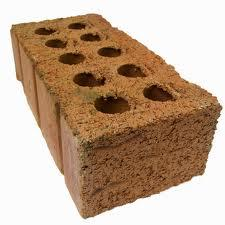Our examples were largely sent or created by pupils and students themselves. Therefore, we would be pleased if you could send us any errors you found, spelling mistakes, or rephasing the example. Thank you!

Leave us a comment of this math problem and its solution (i.e. if it is still somewhat unclear...):Math student
half bricks or half brick?
Or may be better, the weight of a half brick.Dr Math
we updated spelling of this math problem, thank you!Math student
needs rephrasingTips to related online calculators
Do you have a linear equation or system of equations and looking for its solution? Or do you have quadratic equation?

## Next similar math problems:

1. Bent scaleMonica weighed 52 kg. Sara 54 kg. Together they weighed 111 kg. They noticed that the weight on the scale was bent. How much did they really weigh?Adam has half the money in his right pocket than in his left pocket. If he transferred 40 crowns from the left pocket to the right, he would have the same in both pockets. Calculate how many crowns does Adam have in his left pocket more than in his right?
3. Sweets, candyGrandfather gave out sweets to four children. At the last moment, two more children came, so in order to have them all the same, each of the four children would receive four candies less than they would have received if they had not. How much did my grand
4. The testThe test contains four questions, and there are five different answers to each of them, of which only one is correct, the others are incorrect. What is the probability that a student who does not know the answer to any question will guess the right answer
5. Columns of two and threeWhen students in one class stand in columns of two there is none left. When he stands in columns of three, there is one student left. There are 5 more double columns than three columns. How many students are in the class?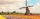How large must the group of people be so that the probability that two people have a birthday on the same day of the year is greater than 90%?
7. ProbabilityHow probable is a randomly selected three-digit number divisible by five or seven?
8. Large familyThe average age of all family members (children, mother, father, grandmother, grandfather) is 29 years. The average age of parents is 40 years, grandparents 66 years and all children are 5 years. How many children are there in this family?
9. Double probabilityThe probability of success of the planned action is 60%. What is the probability that success will be achieved at least once if this action is repeated twice?
10. The orchard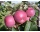4 temporary workers harvested the orchard in 9 days. How many temporary workers we need for six days?
11. TroopsThere are two leaders in the tourist group, with an average age of 30 years and several children with an average age of 10 years. The total age of the group is 12 years. How many children are in the group?
12. Sum of the seventeen numbersThe sum of the 17 different natural numbers is 154. Determine the sum of the two largest ones.
13. Recruitment14. Quiz or testI have a quiz with 20 questions. Each question has 4 multiple choice answers, A, B, C, D. THERE IS NO WAY TO KNOW THE CORRECT ANSWER OF ANY GIVEN QUESTION, but the answers are static, in that if the "correct" answer to #1 = C, then it will always be equal
15. Gold, silver, bronzeHow many ways can we divide gold, silver, bronze medails if there are 6 people competing?
16. The manufacturer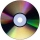The manufacturer found that 3% of the plates produced had a malfunction. Of the compliant, 75% are first and 25% second. What is the probability of producing first and second class plates?
17. Five letters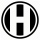How many ways can five letters be arranged?